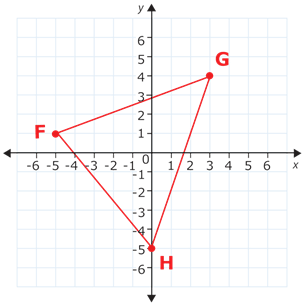# Rational Explorations

Positive numbers are greater than 0. Negative numbers are less than 0.  You can graph positive and negative numbers on a number line.  The positive numbers are to the right of zero and the negative numbers are to the left of 0.  Absolute value is the distance from zero on a number line. Because it refers to a distance, absolute value is always positive.

Opposite numbers are two different numbers that have the same absolute value.

coordinate plane  is formed by two number lines in a plane that intersect at right angles.   The two number lines intersect at 0 on each number line and this point of intersection is called the origin.  The two number lines are called axes.  The horizontal axis is called the x-axis.  The vertical axis is called the y-axis.  The coordinate plane is divided into 4 sections called quadrants.  The x-coordinate tells how far to move right (positive) or left (negative) from the origin.   The y-coordinate tells how far to move up (positive) or down (negative). Just remember, x comes before y in the alphabet, so you will always move along the x-axis first.

## Practice

1)  |-3| = ?

2)  |-5| + |5| = ?

3)  |-7| – |7| = ?

4)  |9  –  4| = ?

5)  | 4 – 9 | = ?

6)  Name the coordinates.7)  Name the coordinates.8)  Print graph paper or draw vertical lines on lined notebook paper Plot these points.

• (-3, 3)
• (-5, 3)
• (1, 4)
• (2, -3)

(source)